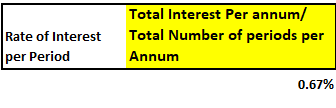### How To Calculate Loan Payoff In Excel

Loan payoffs are simple calculations that is required every time you take a loan from a bank or a financial institution. To prevent yourself from being cheated by others or to be able to help others with their loans, follow the steps below for the loan payoff calculation.

Step 1: Input the details of Loan Amount, number of years of loan repayment, Rate of Interest, and Period of Loan payment

• Loan Amount: Principal amount borrowed
• Years of Loan repayment: Total no. of years in which loan is completely repaid
• Rate of Interest: Per annum interest on the principal amount
• Period: The span during which the loan payment is made (Ex: Each month, each quarter etc.)Here, No. of Periods = No. of Years * 12 (monthly repayment) = 10*12

Note: You can choose shorter loan amortization period eg. 6, 12 or 30 months instead of 10 years.

Step 2: Calculate the Rate of interest per period.

For above figures, rate of interest per period = (8/12) % = 0.67%Step 3: Calculate the loan payoff per period using excel PMT formula.

=PMT( Rate, Nper, Pv, Fv, Type)

• Rate: Per period interest rate –
• Nper: Total no. of periods – Pv: Initial Value of the loan (Has to be negative for PMT formula) –
• Fv (optional): Fv stands for future value. If after paying off the last loan payment, you want to have any balance left it is the future value. Fv can be omitted (considered 0 if omitted) –
• Type (optional)

0: If payment is to be done at end of period

1: If payment is to be done at beginning of periodLoan payoff can be simply calculated by using the PMT formula and using the correct variables.Full formula is =PMT(E8,E6,-E4,0)

Good luck with paying off your loans.

## Template

```Further reading: